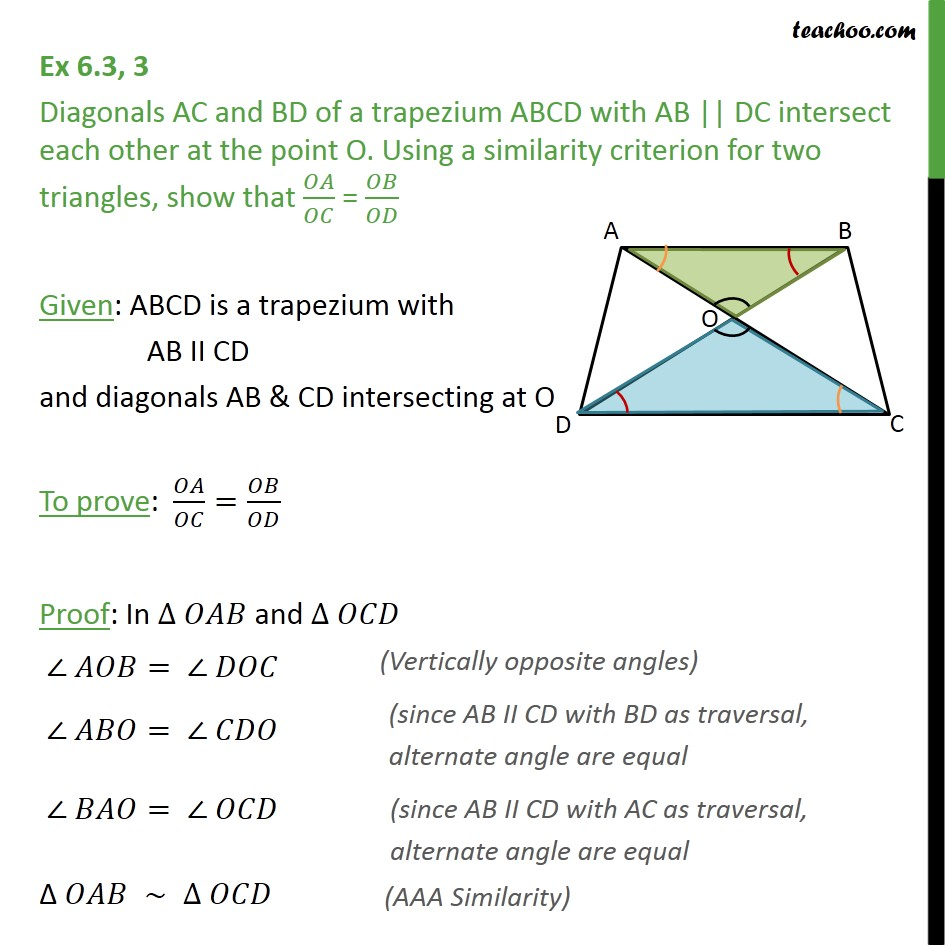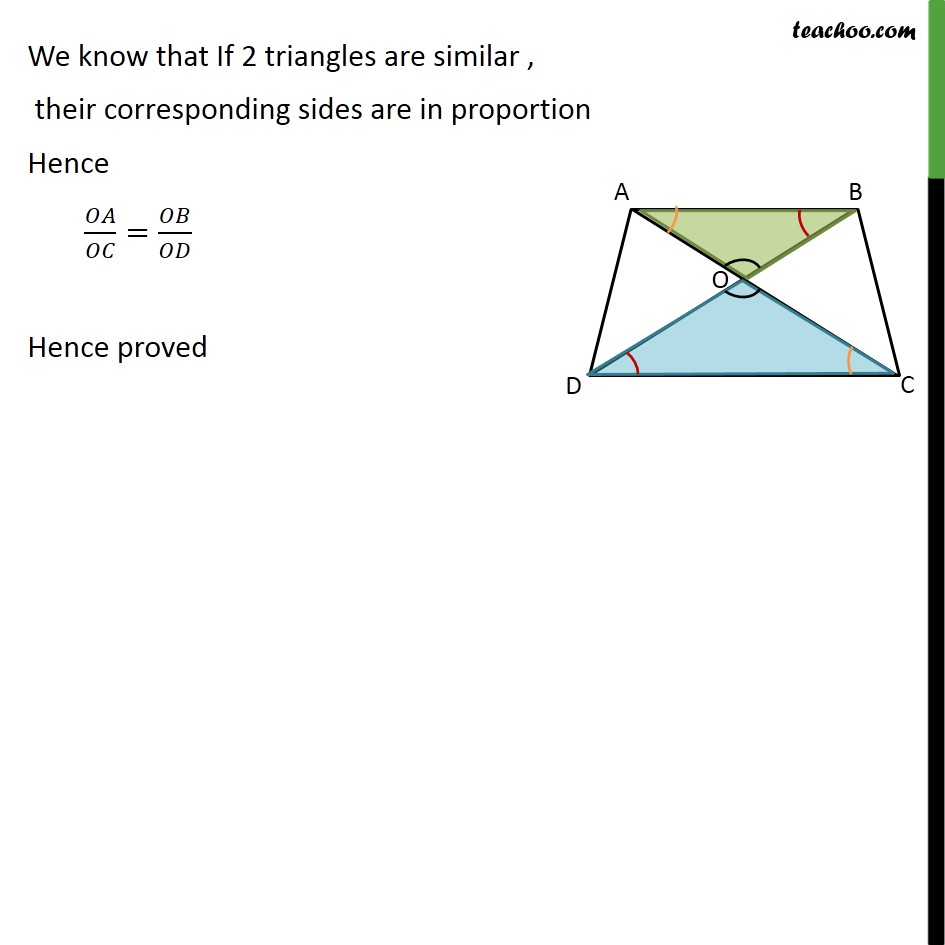Ex 6.3

Chapter 6 Class 10 Triangles
Serial order wiseGet live Maths 1-on-1 Classs - Class 6 to 12

### Transcript

Ex 6.3, 3 Diagonals AC and BD of a trapezium ABCD with AB || DC intersect each other at the point O. Using a similarity criterion for two triangles, show that / = / Given: ABCD is a trapezium with AB II CD and diagonals AB & CD intersecting at O To prove: / = / Proof: In and = = = We know that If 2 triangles are similar , their corresponding sides are in proportion Hence / = / Hence proved